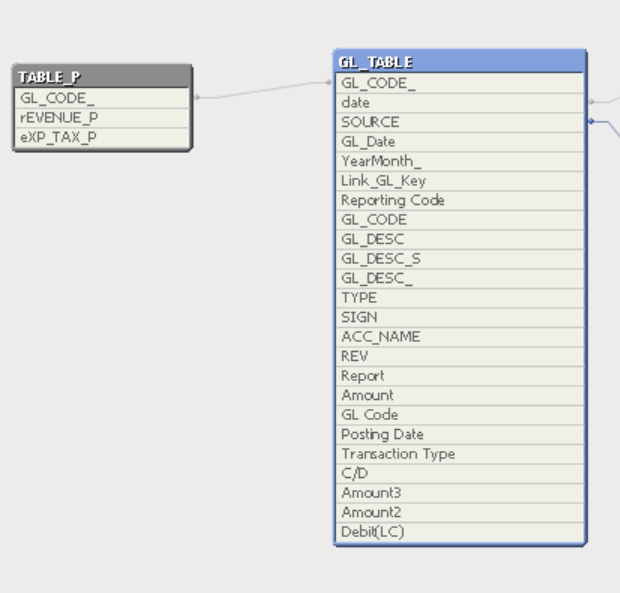# QlikView App Dev

Discussion Board for collaboration related to QlikView App Development.

Announcements
Join this live chat April 6, 10AM EST - QlikView to Qlik Sense REGISTER
cancel
Showing results for
Search instead for
Did you mean:Master

## My partial reload cannot load 2 field

Hi All

i Have 2 partial load script

Part (1) work fine :-

TABLE_P:
left keep (GL_TABLE)
load GL_CODE_,

If([GL_CODE_]>=5000001 and [GL_CODE_]<=5999999,'rEVENUE_P') as [rEVENUE_P],
If([GL_CODE_]>=9400020 and [GL_CODE_]<=9400020,'eXP_TAX_P') as [eXP_TAX_P]

resident GL_TABLE;

Part (2) Not working ( After i load [Reporting Code] field name )

TABLE_P:
left keep (GL_TABLE)
load GL_CODE_,[Reporting Code],

If([GL_CODE_]>=5000001 and [GL_CODE_]<=5999999,'rEVENUE_P') as [rEVENUE_P],
If([GL_CODE_]>=9400020 and [GL_CODE_]<=9400020,'eXP_TAX_P') as [eXP_TAX_P]

resident GL_TABLE;

May i know how to solve it ? if i still need to load 2 field.

Part 2 Script , now working data model :-Part (1) Script  data model Below is working fine :-enclosed my QVW which is working fine , but i need to add one more field Reporting Code at partial load.

Hope some one can advise me where go wrong ?

Paul

0 Replies